# NEET Physics Motion in A Straight Line Questions Solved

A ball is thrown upward with a certain speed. It passes through the same point at 3 second and 7 second from the start. The maximum height achieved by the ball is

1.  500 m

2.  250 m

3.  125 m

4.  450 m

(3)

As upward and downward motion are symmetric [Time taken from A$\to$B is same as time taken from B$\to$C]

So time to reach highest point is 5s. Same time is required to reach from B to ground.

Time to reach highest point after 3sec = $\frac{7-3}{2}=2sec.$

Time to reach highest point from ground = 3+2 = 5sec.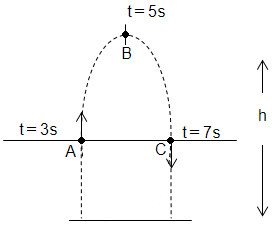For downward motion,

Difficulty Level:

• 17%
• 26%
• 53%
• 5%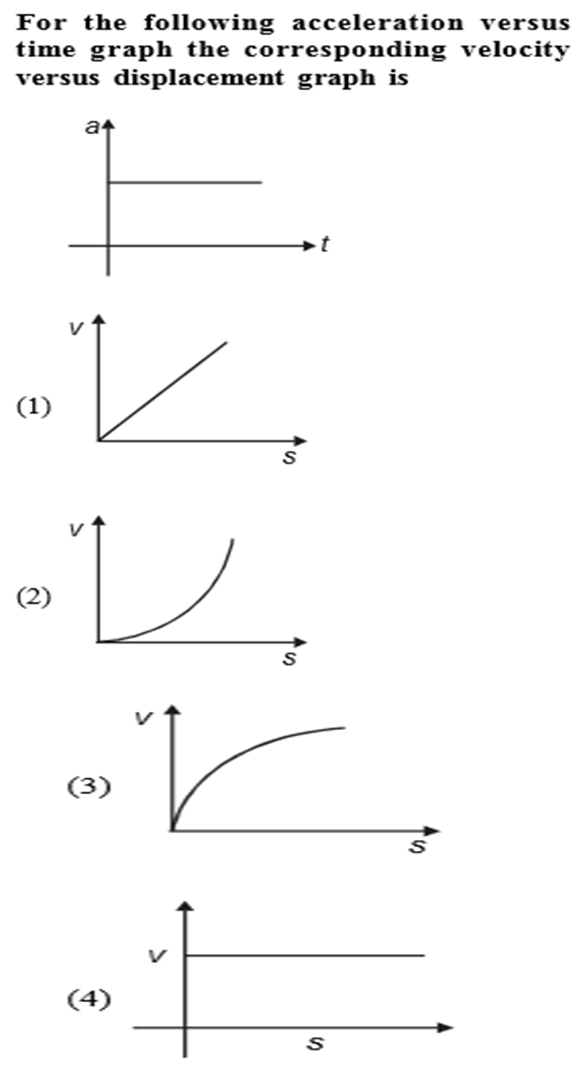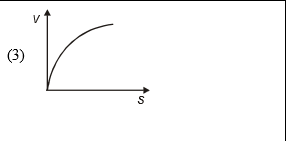$a=\frac{dv}{dt}=\frac{dv}{ds}×\frac{ds}{dt}=v×\frac{dv}{ds}$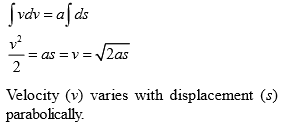Difficulty Level:

• 43%
• 21%
• 23%
• 15%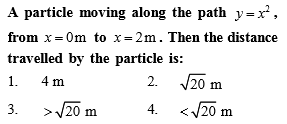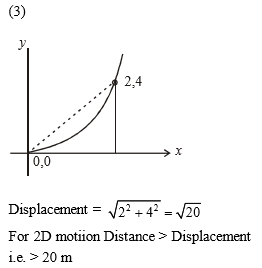Difficulty Level:

• 56%
• 18%
• 24%
• 4%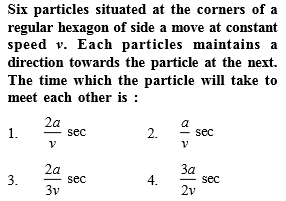(1)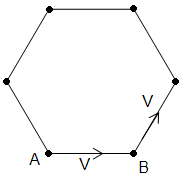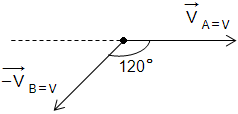Difficulty Level:

• 42%
• 30%
• 22%
• 9%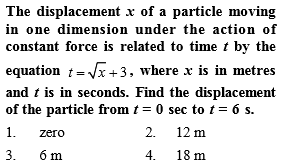(1)

Difficulty Level:

• 58%
• 15%
• 14%
• 15%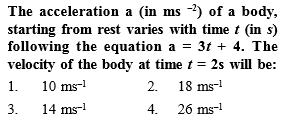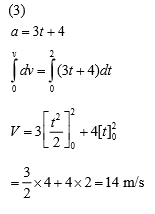Difficulty Level:

• 22%
• 13%
• 64%
• 3%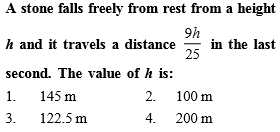Distance travelled in t second,

Difficulty Level:

• 15%
• 19%
• 62%
• 6%

A point moves in a straight line under the retardation a${\mathrm{v}}^{2}$. If the initial velocity is u, the distance covered in 't' seconds is-

1.  $\mathrm{aut}$

2.

3.

4.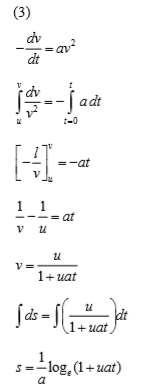Difficulty Level:

• 17%
• 27%
• 51%
• 7%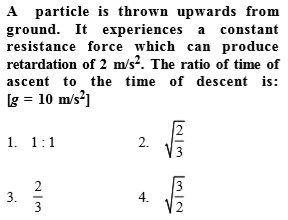(2)

Upward journey                                                                   Downward journey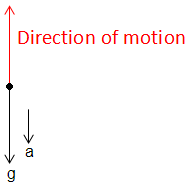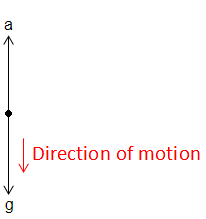Net acceleration = -(a+g)                                          Net acceleration = g - a
-ve indicates retardation

Difficulty Level:

• 40%
• 36%
• 16%
• 10%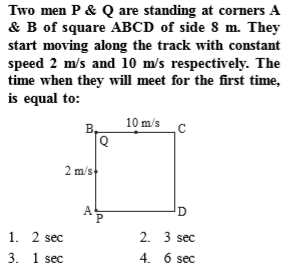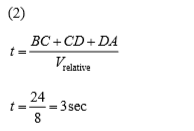Difficulty Level:

• 15%
• 51%
• 27%
• 9%